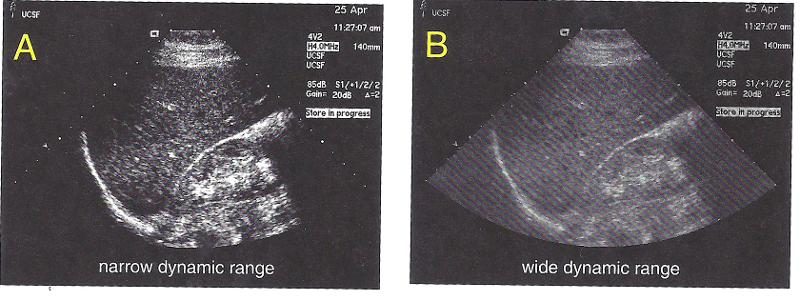# Understanding Ultrasound Physics: Chapter 16 Dynamic Range Flashcards

Set Details Share
created 9 years ago by Annabelle
1,286 views
updated 9 years ago by Annabelle
Page to share:
Embed this setcancel
COPY
code changes based on your size selection
Size:
X
1

Dynamic Range

a method of describing the extent to which a signal can vary and still be processed

2

Dynamic range general rule

the dynamic range of information decreases the more it is processed

3

transducer dynamic range

120 dB

4

100 to 120 dB

5

scan converter dynamic range

40 to 50 dB

6

display dynamic range

20 to 30 dB

7

archive dynamic range

10 to 30 dB

8

How to calculate a component's dynamic range

1. divide the bigger voltage by the smaller voltage
2. count the # of zeros in the number - for each zero there is 20 dB more dynamic range

9

dynamic range

10:1

20 dB

10

dynamic range

100:1

40 dB

11

dynamic range

1,000:1

60 dB

12

dynamic range

10,000:1

80 dB

13

dynamic range

100,000:1

100 dB

14

A range from 1 volt to 100 volts can be processed accurately by the display of an ultrasound system. What is the dynamic range of the display?

40 dB

15

A component of an ultrasound system can properly process voltages ranging from 1 mV to 1 V. What is the dynamic range?

60 dB

16

A component of an ultrasound system can properly process voltages ranging from 5 V to 5000 V. What is the dynamic range?

60 dB

17

The same mathematics technique is used to calculate the gain of a component when the signal has units of volts.

An amplifier increases a voltage from .2 to 2000 volts. How many decibels of gain in this?

80 dB

18

An amplifier increases a voltage from 5 to 500 volts. How many decibels of gain is this?

40 dB

19

Compression

technique that reduces the dynamic range of a signal without introducing errors

20

requirements of compression

largest signal remains the largest

smallest signal remains the smallest

the range of signals is reduced

21

An uncompressed signal within the receiver of an ultrasound system has a dynamic range of 110 dB. The signal undergoes 40 dB of compression. What is the dynamic range of the compressed signal?

70 dB

110 dB - 40 dB = 70 dB

22

An uncompressed signal has a dynamic range of 85 dB. The signal undergoes 30 dB of compression. What is the dynamic range of the compressed signal?

55 dB

85 dB - 30 dB = 55 dB

23

After compression, a signal within the ultrasound system has dynamic range of 70 dB. The original signal was compressed by 40 dB. What is the dynamic tange of the original, uncompressed signal?

110 dB

70 dB + 40 dB = 110 dB

24

A signal within the system's receiver has a dynamic range of 60 dB after compression. The original signal was compressed by 50 dB. What is the dynamic range of the original uncompressed signal

110 dB

60 dB + 50 dB = 110 dB

25

number of choicesdynamic range can report the number of possible gray shades.

26

Narrow dynamicfew choices
black and white (bistable)
high contrast

27

Wide dynamic range